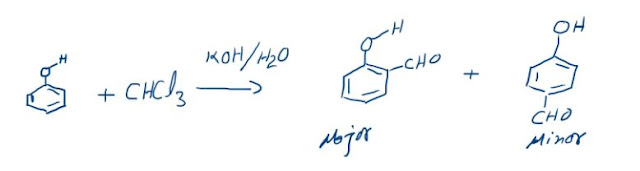## Fajan rule

### Change of Ionic Character to Covalent Character

When two oppositely charged ions of unequal size approach each other closely (during formation of an electrovalent bond), the ion smaller in size attracts outermost electrons of the other ion and repel its nuclear charge. The net result is distortion or polarisation of the bigger ion. This distortion is usually done by the cation as its size is smaller than the anion.
The electron cloud of anion no longer remains symmetrical but is elongated towards the cation. The ability of a cation to polarise the nearby anion is called its Polarising power and the tendency of an anion to get distorted or deformed or polarised by the cation is called its Polarisability. Due to polarisation, sharing of electrons occur between two ions to some extent and the bond shows some covalent character.
This is shown in figure.
The magnitude of polarisation depends upon a number of factors or the increased covalent character is favoured by a number of factors. These factors were suggested by Fajan and are known as Fajan’s rule:

A) Small Positive Ion (Cation): Due to greater concentration of positive charge on a small area, the smaller cation has high polarising power. This explains why LiCl is more covalent than KCl.

B) Large Negative Ion (Anion): The larger the anion, the greater is its Polarisability, i.e. susceptibility to get polarised. It is due to the fact that the outer electrons of a large anion are loosely held and hence can be more easily pulled out by the cation. This explains why iodides, among halides, are most covalent in nature.

C) Large Charge on Either of the Two Ions: As the charge on the ion increases, the electrostatic attraction of the cation for the outer electrons of the anion also increases, with the result its ability for forming the covalent bond increases.

D) Electronic Configuration of the Cation:  For the two ions of the same size and charge, one with a pseudo noble gas configuration (i.e., 18 electrons in outer-most shell) than a cation with noble gas configuration (i.e. 8 electrons in outermost shell) will be more polarising. Thus copper (I) chloride is more covalent than sodium chloride although Cu+ ion (0.96A°) and Na+ ion (0.95A°) have same size and charge.
LiF  = 870°C   LiCl = 613°C
LiBr = 547°C   LiI   = 446°C

From the above discussion, we find that greater the possibility of polarisation, lower is the melting point and heat of sublimation and greater is the solubility in non-polar solvents.

Percentage of Ionic Character
Every ionic compound having some percentage of covalent character according to Fajan’s rule. The percentage of ionic character in a compound having some covalent character can be calculated by the following equation.

% ionic character = Obs. dipole moment/ Calc. dipole moment × 100

Example 1: Dipole moment of KCl is 3.336 × 10–29 coulomb metre which indicates that it is highly polar molecule. The interatomic distance between k+ and Cl is 2.6 ×10–10 m. Calculate the dipole moment of KCl molecule if there were opposite charges of one fundamental unit located at each nucleus. Calculate the % ionic character of KCl.

Solution:
Dipole moment μ = e × d coulomb metre
For KCl d = 2.6 × 10–10 m
For complete separation of unit charge
e = 1.602 × 10–19 C
Hence μ = 1.602 × 10–19 × 2.6 × 10–10 = 4.1652 × 10–29 Cm
μ KCl = 3.336 × 10–29 Cm
∴ % ionic character of KCl = 3.336×10–29/4.165×10–29 x 100= 80.09%

Example 2. Calculate the % of ionic character of a bond having length = 0.83 Å and 1.82 D as it’s observed dipole moment.
Solution: To calculate μ considering 100% ionic bond
= 4.8 × 10–10 × 0.83 × 10–8 esu cm
= 4.8 × 0.83 × 10–18 esu cm = 3.984 D
∴ % ionic character = 1.82/3.984 × 100 = 45.68 %
The % ionic character is nearly 43.25%,   so the
% covalent character is (100 – 43.25) = 56.75%.

From the octet rule HF should have been a purely covalent compound but actually it has some amount of ionic character in it, which is due to the electronegativity difference of H and F. Similarly knowing the bond length and observed dipole moment of HCl, the % ionic character can be known. It was found that HCl has 17% ionic character. Thus it can be clearly seen that although we call HCl and HF as covalent compounds but it has got appreciable amount of ionic character. So from now onwards we should call a compound having more of ionic less of covalent and vice versa rather than fully ionic or covalent.

### The Reimer-Tiemann reaction: Reaction and its Mechanism

Reimer-Tiemann Reaction Phenols on reaction with chloroform in the presence of sodium hydroxide (or potassium hydroxide) solution give hydro...